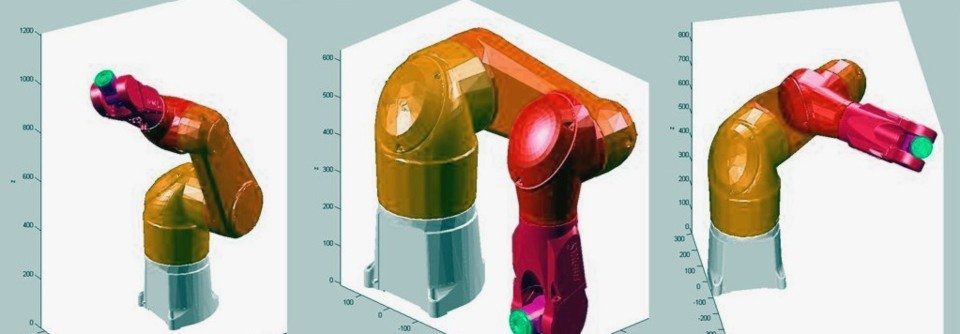# When all else fails... Matlab

For the ME4444 Senior Design project, a mathematical model was needed to understand the lamina flow engine. Because the heat input is continuous and a function of the pressure and temperature of the hot chamber, a numerically iterative model was developed. The program functions by calculating the change in entropy, enthalpy, temperature, pressure, and energy as the stroke moves a few degrees. The following are data outputs.

Heat Lag Analysis
Lamina_Flow_Engine_Thermodynamic_Cycle_V[...]